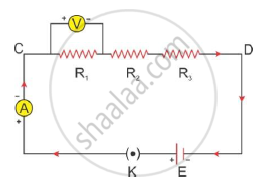# Find the Expression for the Resistors Connected in Series and Write the Two Characteristics of It. - Science and Technology 1

Find the expression for the resistors connected in series and write the two characteristics of it. (Draw figure).

#### Solution

Expression for the resistors in series:R1, R2 and R3 are the three resistors connected in series between points C and D as shown in the figure above. I is the current and V is the PD across points

C and D.

Rs is the effective resistance in a circuit, and V1, V2 and V3 are the potentials across the three resistors such that
V = V1 + V2 + V3 ---- (1)

Using Ohm’s law, the total potential difference
V = I Rs
and V1 = IR1, V2 = IR2 and V3 = IR3

Substituting these values in Equation 1, we get

IRs = IR1 + IR2 + IR3
∴ Rs = R1 + R2 + R3
For n number of resistors connected in series,
Rs = R1 + R2 + R3 + R4 + ----- + Rn

Characteristics of a series combination of resistors:
a) The same current flows through each resistor.
b) The effective resistance of the combination is greater than any of the resistances in the combination.
c) The effective resistance of the combination is equal to the sum of individual resistors.

Concept: System of Resistors - Resistors in Series
Is there an error in this question or solution?
2015-2016 (March)

Share